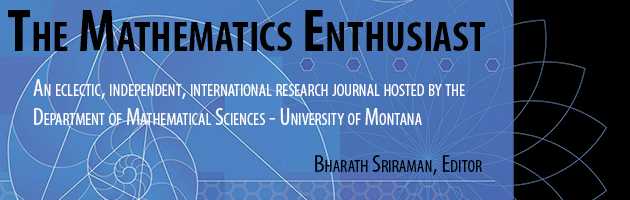•
•5

1

#### Abstract

This paper explores how the constructions of mathematically gifted fifth and sixth grade students using Euler’s polyhedron theorem compare to those of mathematicians as discussed by Lakatos (1976). Eleven mathematically gifted elementary school students were asked to justify the theorem, find counterexamples, and resolve conflicts between the theorem and counterexamples. The students provided two types of justification of the theorem. The solid figures suggested as counterexamples were categorized as 1) solids with curved surfaces, 2) solids made of multiple polyhedra sharing points, lines, or faces, 3) polyhedra with holes, and 4) polyhedra containing polyhedra. In addition to using the monster-barring method, the students suggested two new types of conjectures to resolve the conflicts between counterexamples and the theorem, the exception-baring method and the monster-adjustment method. The students’ constructions resembled those presented by mathematicians as discussed by Lakatos.

125

142

COinS